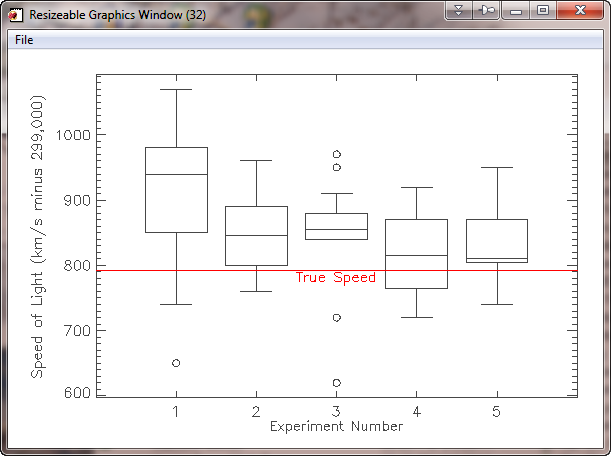# Drawing a Box and Whisker Plot

QUESTION: Can you show me how to draw a box and whisker plot in IDL. The box on the plot should be drawn around the 25th and 75 quartile of the data, and the whiskers should extend out to largest and smallest value within 1.5 times the interquartile range (IGR). Outliers should be marked with circles.ANSWER: The idea behind a box and whisker plot is that the data should first be divided into two equal groups by finding the median value of the data. Then, each of these two sub-groups should be divided in the same way. If done properly, this should divide the data into four equally populated sub-groups. The divisions between groups are called the 25th quartile, the median value, and the 75th quartile.

In the cgBoxplot program I wrote to do this, I sort the data and find the quartiles and the IRG like this.

```   sortedData = data[Sort(data)]
IF N_Elements(sortedData) MOD 2 EQ 0 THEN BEGIN
index = N_Elements(sortedData)/2
medianData = (sortedData[index-1] + sortedData[index]) / 2.0
lowerGroup = sortedData[0:index-1]
higherGroup = sortedData[index:N_Elements(data)-1]
ENDIF ELSE BEGIN
index = N_Elements(sortedData)/2
medianData = sortedData[index]
lowerGroup = sortedData[0:index-1]
higherGroup = sortedData[index+1:N_Elements(data)-1]
ENDELSE
quartile_25 = Median(lowerGroup, /EVEN)
quartile_75 = Median(higherGroup, /EVEN)
irq = quartile_75 - quartile_25
```

The next step is easy. All we have to do is use IDL's graphics commands to draw lines and symbols on a plot. Given that we have a width of the box and a location where we should draw the box along the X axis (in the variables width and xlocation, resprectively), we can draw the box plot like this. Note how I use Value_Locate to identify those data that are inside the part of the plot represented by the whiskers.

```   minData = MIN(data, MAX=maxData)
halfwidth = width / 2.0
x1 = xlocation - halfwidth
x2 = xlocation + halfwidth
y1 = quartile_25
y2 = quartile_75
cgPlotS, [x1,x1,x2,x2,x1], [y1,y2,y2,y1,y1], COLOR=color
cgPlotS, [x1, x2], [medianData, medianData], COLOR=color

; Are there any data greater than 1.5*irq
imax = Where(data GT quartile_75 + (1.5 * irq), maxcount)
IF maxcount EQ 0 THEN BEGIN
top = maxData
ENDIF ELSE BEGIN
index = Value_Locate(sortedData, quartile_75 + (1.5 * irq))
top = sortedData[0 > (index) < (N_Elements(data)-1)]
ENDELSE

; Are there any data less than 1.5*irq
imin = Where(data LT quartile_25 - (1.5 * irq), mincount)
IF mincount EQ 0 THEN BEGIN
bottom = minData
ENDIF ELSE BEGIN
index = Value_Locate(sortedData, quartile_25 - (1.5 * irq))
bottom = sortedData[0 > (index+1) < (N_Elements(data)-1)]
ENDELSE

; Draw the whiskers.
cgPlotS, [xlocation, xlocation], [quartile_75, top], COLOR=color
cgPlotS, [xlocation, xlocation], [quartile_25, bottom], COLOR=color
cgPlotS, [xlocation - (halfwidth*0.5), xlocation + (halfwidth*0.5)], \$
[top, top], COLOR=color
cgPlotS, [xlocation - (halfwidth*0.5), xlocation + (halfwidth*0.5)], \$
[bottom, bottom], COLOR=color

; Draw outliners if there are any.
IF maxcount GT 0 THEN BEGIN
FOR j=0,maxcount-1 DO cgPlotS, xlocation, data[imax[j]], \$
PSYM=cgSymCat(9), COLOR=color
ENDIF
IF mincount GT 0 THEN BEGIN
FOR j=0,mincount-1 DO cgPlotS, xlocation, data[imin[j]], \$
PSYM=cgSymCat(9), COLOR=color
ENDIF
```

As an example, you can download data from the Michaelson-Morely experiment in which they measured the speed of light. The data is in a file named mm_data.dat. You can use this code to open and read the data in the file, and display it as a box plot.

```   OpenR, 1, 'mm_data.dat'
data = Intarr(5, 20)
Close, 1
cgBoxPlot, data, XTITLE='Experiment Number', \$
YTITLE='Speed of Light (km/s minus 299,000)', /Window
cgText, 3, 775, /Data, Color='red', 'True Speed', Alignment=0.5, /AddCmd
```

You can see the results in the figure below.A box and whisker plot in IDL using data from the Michaelson-Morley experiment.

A different version of this plot can be found in the Coyote Plot Gallery.Web Coyote's Guide to IDL Programming